## LetsPlayMaths.Com

WELCOME TO THE WORLD OF MATHEMATICS

# Class 6 Geometry

Introduction to Geometry

Point

Line Segment

Line

Ray

Opposite Rays

Intersecting Lines

Parallel Lines

Concurrent Lines

Collinear Points

Rectangle

Square

Parallelogram

Rhombus

Circle

Geometry Test

Geometry Worksheet

## Introduction to Geometry

The word geometry comes from two Greek words, namely Geo and Metron. Here Geo means earth and metron means measurement. So the word geometry means, measurement of earth.

## Point

A point is a mark of position, it has neither length nor breadth nor depth. We denote a point by a capital letter A, B, C, D, etc. as shown in the below figure.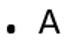## Line Segment

Let us consider two points P and Q as shown in the below figure. The straight path from P to Q is known as line segment PQ.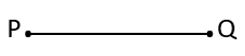PQ = QP

Two line segments AB and CD are said to be congruent, if their lengths are equal and we write, AB = CD.

We use a ruler to measure the length of a line segment. One edge of the ruler is marked in centimeters and millimeters, other edge of the rules is marked in inch.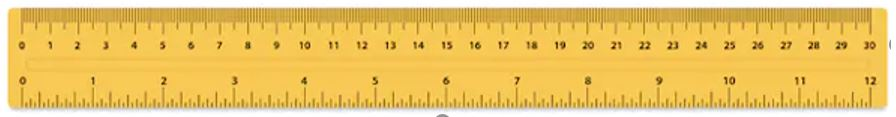Place the edge of the ruler along the given line PQ, keeping the 0 cm mark of the ruler at the point P, as shown below.Read the mark on the ruler against the point Q. In this case Q is on 9 cm. So, the length of PQ is 9 cm.

## Line

A line segment extended endlessly on both sides is known as Line. Picture below shows a example of line.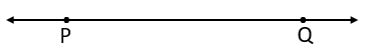A line has no end points and it does not have a definite length. Sometimes, we denote a line by a small letter p, q , r, etc.

## Ray

A line segment extended endlessly in one direction is known as ray. It has only one end point and can be extended indefinitely in one direction only.

Let's draw a ray with initial point 'O' and passing through a given point 'P'.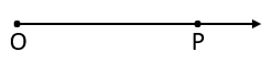Ray OP and ray PO are not same they are the opposite rays. Ray OP extends indefinitely in the direction from O to P, while ray PO extends indefinitely in the direction from P to O.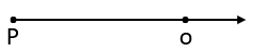A ray has no definite length.

## Opposite Rays

Two rays having same initial point and extending indefinitely in opposite directions are known as opposite rays.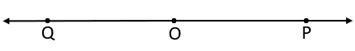In the above figure, OP and OQ are two rays with the same initial point 'O' and extending indefinitely in opposite directions along the same lines. So, OP and OQ are opposite rays.

## Intersecting Lines

If there is a point 'x' common to two lines 'p' and 'q' in the same plane, we can say that the two lines are intersecting at point 'x'. This point x is known as point of intersection.## Parallel Lines

Two lines are called parallel lines if they do not intersect at all. The distance between two parallel lines always remains same. Railway tracks are best example parallel lines.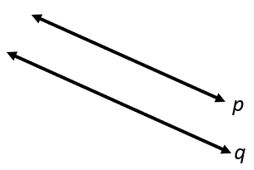## Concurrent Lines

Three or more lines are said to be concurrent lines if all the lines pass through the same point. The common point is known as point of concurrence of the given lines.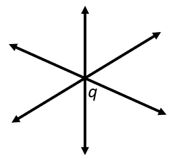## Collinear Points

Three or more points in a plane are called Collinear points if and only if all of them lie on the same line.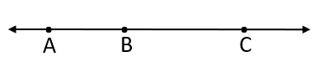Any figure formed by four line segments is known as quadrilateral. In the below given figure ABCD is a quadrilateral.It has four sides, they are AB, BC, CD and DA.

It has four vertices, they are A, B, C and D.

There are various types of quadrilaterals.

1. Rectangle
2. Square
3. Parallelogram
4. Rhombus

## Rectangle

A quadrilateral having equal opposite sides and all four angles are 90° then it is known as a rectangle.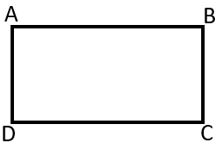In the above rectangle AB = CD and BC = AD

∠A = ∠B = ∠C = ∠D = 90°

Add opposite vertices with lines AC and BD as shown in below figure. Both AC and BD are known as diagonals.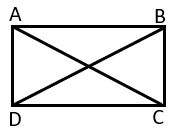A rectangle can have maximum two diagonals.
AC = BD

## Square

A quadrilateral having all sides and angles(90°) are equal is known as square.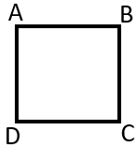In the above square AB = BC = CD = AD and ∠A = ∠B = ∠C = ∠D = 90°

Add opposite vertices with lines AC and BD as shown in below figure. Both AC and BD are known as diagonals.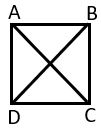A square can have maximum two diagonals.

AC = BD

## Parallelogram

A quadrilateral having below mentioned properties are known as parallelogram.

1. Opposite sides are equal
2. Opposite sides are parallel
3. Opposite angles are equal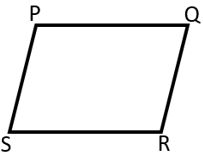## Rhombus

A parallelogram having all sides equal is known as rhombus.AB || CD, BC ||AD and AB = BC = CD = AD

## Circle

A circle is the collection of all the points in a plane that are present at a constant distance from a fixed point. This fixed point is called the centre and the constant distance is called the radius of the circle.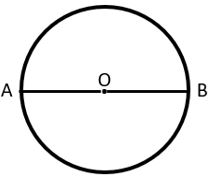Here 'O' is the centre of the circle.
AO and OB are the radius of the circle. OA = OB = r
AB is the diameter of the circle and it is twice the length of the radius. Let's consider diameter as 'd'

d = 2 x r

## Class-6 Geometry Test

Geometry Test - 1

Geometry Test - 2

## Class-6 Geometry Worksheet

Geometry Worksheet - 1

Geometry Worksheet - 2

Geometry Worksheet - 3